知识图谱与机器学习 ｜ KG入门 -- Part1-b 图深度学习

图深入学习越来越重要。在这里，我将使用Spektral库和平台MatrixDS展示关于图的机器学习和深度学习的基本思想。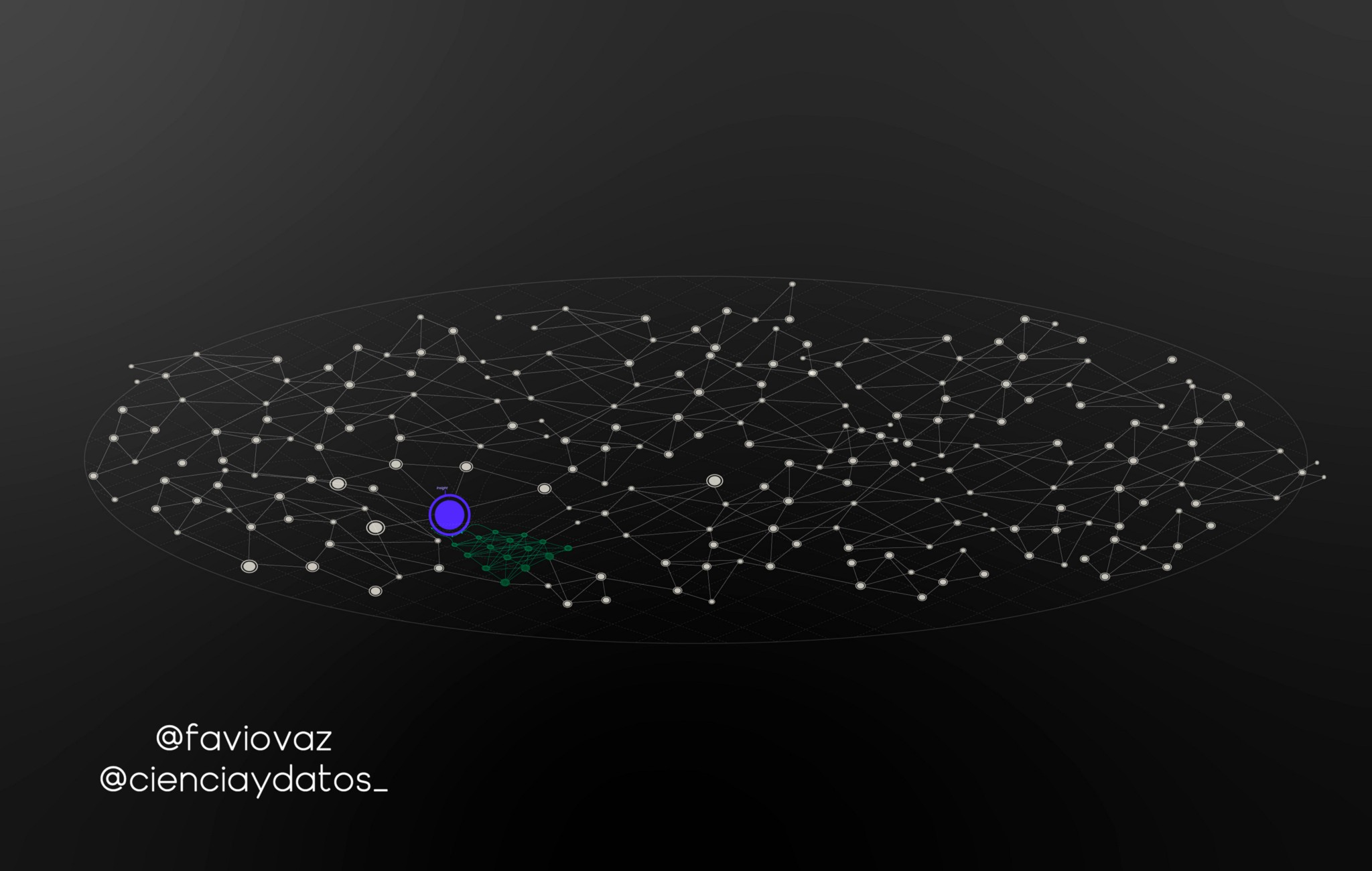编译 | 魔图君与Arno 来源 | Medium

介绍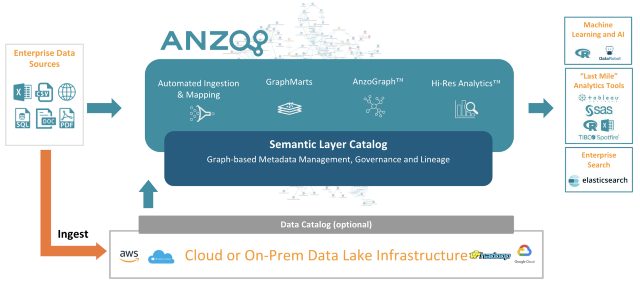目标

细节

• 描述图深度学习的基础
• 探索Spektral库
• 验证对Data Fabric进行深度学习的可能性。

第一节 图深度学习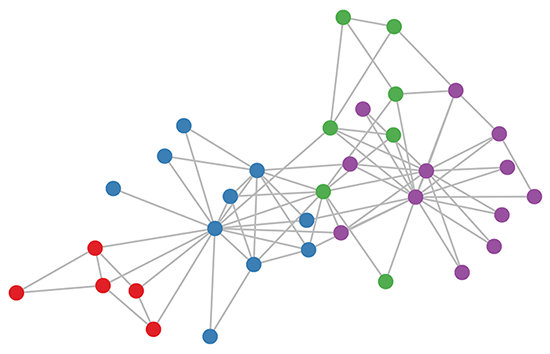Spektral库的文档中声明图一般由三个矩阵表示:

• A $\in${0,1} ^ {N$\times$N}:一种二值邻接矩阵，如果节点i与j之间有连接，则$A_{ij}$=1，否则$A_{ij}$=0;
• X $\in$ R ^ (N$\times$F): 编码节点属性(或特征)的矩阵，其中F维属性向量与每个节点相关联;
• E $\in$ R ^ (N$\times$N$\times$S)：一种编码边属性的矩阵，其中一个s维属性向量与每个边相关联。

https://towardsdatascience.com/how-to-do-deep-learning-on-graphs-with-graph-convolutional-networks-7d2250723780

图神经网络(GNN)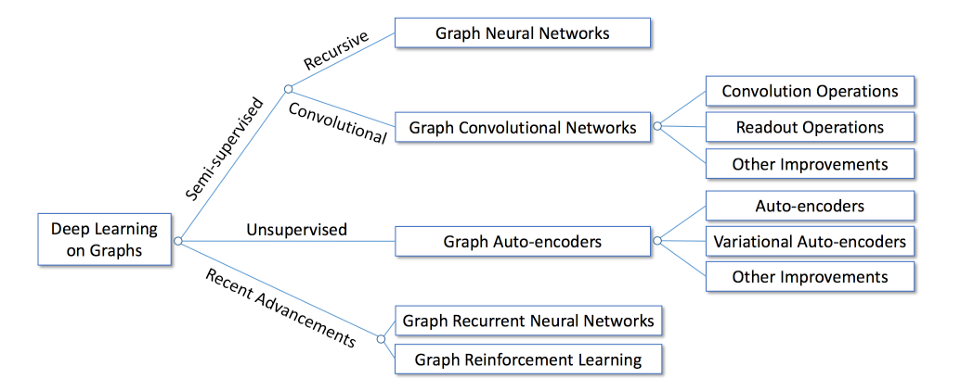GNN的思想很简单:为了对图的结构信息进行编码，每个节点$v_i$可以表示为一个低维状态向量$s_i$, 1≤i≤N(记住向量可以看作秩为1的张量，张量可以用矩阵表示)。

第二节 使用Spektral进行深度学习Spektral作者将Spektral定义为关系表示学习的框架，用Python构建并基于Keras API。

安装

https://community.platform.matrixds.com/community/project/5c6ae7c8c1b06ba1e18f2a6e/files

数据表示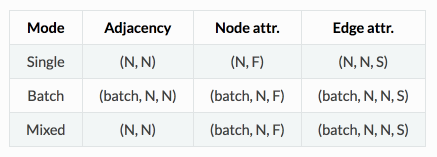from spektral.datasets import citation

Out: (2708, 2708)

In : node_attributes.shape
Out: (2708, 2708)

In : edge_attributes.shape
Out: (2708, 7)

使用图注意层(GAT)进行半监督分类

https://community.platform.matrixds.com/community/project/5c6ae7c8c1b06ba1e18f2a6e/files

GAT是一种新型的神经网络结构，它利用掩蔽的自注意层对图形结构数据进行操作。在Spektral中，GraphAttention层计算卷积与layers.GraphConv类似，但是使用注意机制来加权邻接矩阵，而不是使用归一化拉普拉斯。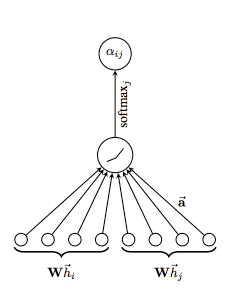# Layers
dropout_1 = Dropout(dropout_rate)(X_in)
graph_attention_1 = GraphAttention(gat_channels,
dropout_rate=dropout_rate,
activation='elu',
kernel_regularizer=l2(l2_reg),
attn_kernel_regularizer=l2(l2_reg))([dropout_1, A_in])
dropout_2 = Dropout(dropout_rate)(graph_attention_1)
graph_attention_2 = GraphAttention(n_classes,
dropout_rate=dropout_rate,
activation='softmax',
kernel_regularizer=l2(l2_reg),
attn_kernel_regularizer=l2(l2_reg))([dropout_2, A_in])
# Build model
model = Model(inputs=[X_in, A_in], outputs=graph_attention_2)
model.compile(optimizer=optimizer,
loss='categorical_crossentropy',
weighted_metrics=['acc'])
model.summary()
# Callbacks
es_callback = EarlyStopping(monitor='val_weighted_acc', patience=es_patience)
tb_callback = TensorBoard(log_dir=log_dir, batch_size=N)
mc_callback = ModelCheckpoint(log_dir + 'best_model.h5',
monitor='val_weighted_acc',
save_best_only=True,
save_weights_only=True)

__________________________________________________________________________________________________
Layer (type)                    Output Shape         Param #     Connected to
==================================================================================================
input_1 (InputLayer)            (None, 1433)         0
__________________________________________________________________________________________________
dropout_1 (Dropout)             (None, 1433)         0           input_1
__________________________________________________________________________________________________
input_2 (InputLayer)            (None, 2708)         0
__________________________________________________________________________________________________
graph_attention_1 (GraphAttenti (None, 64)           91904       dropout_1
input_2
__________________________________________________________________________________________________
dropout_18 (Dropout)            (None, 64)           0           graph_attention_1
__________________________________________________________________________________________________
graph_attention_2 (GraphAttenti (None, 7)            469         dropout_18
input_2
==================================================================================================
Total params: 92,373
Trainable params: 92,373
Non-trainable params: 0

# Train model
y_train,
epochs=epochs,
batch_size=N,
validation_data=validation_data,
shuffle=False,  # Shuffling data means shuffling the whole graph
callbacks=[es_callback, tb_callback, mc_callback])

print('Evaluating model.')
y_test,
batch_size=N)
print('Done.\n'
'Test loss: {}\n'
'Test accuracy: {}'.format(*eval_results))

https://community.platform.matrixds.com/community/project/5c6ae7c8c1b06ba1e18f2a6e/files

第三节 这在Data Fabric中处于什么位置?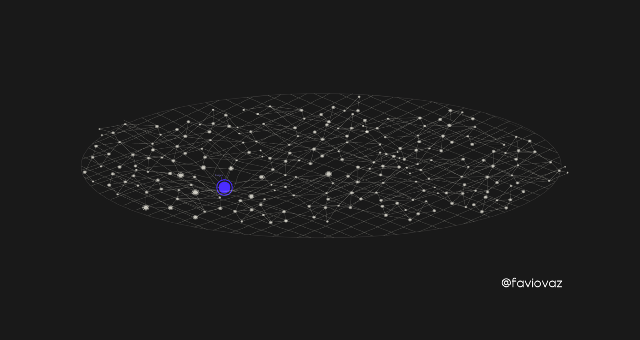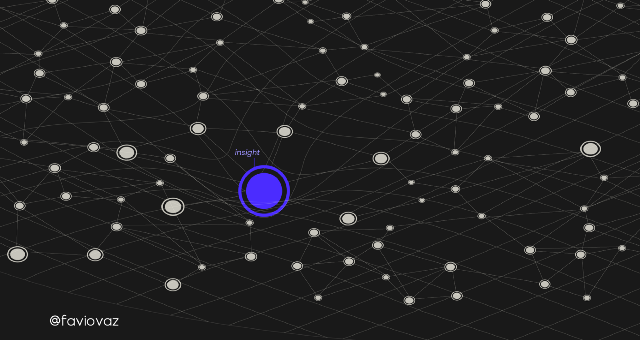ind.dataset_str.x => the feature vectors of the training instances as scipy.sparse.csr.csr_matrix object;    ind.dataset_str.tx => the feature vectors of the test instances as scipy.sparse.csr.csr_matrix object;    ind.dataset_str.allx => the feature vectors of both labeled and unlabeled training instances        (a superset of ind.dataset_str.x) as scipy.sparse.csr.csr_matrix object;    ind.dataset_str.y => the one-hot labels of the labeled training instances as numpy.ndarray object;    ind.dataset_str.ty => the one-hot labels of the test instances as numpy.ndarray object;    ind.dataset_str.ally => the labels for instances in ind.dataset_str.allx as numpy.ndarray object;    ind.dataset_str.graph => a dict in the format {index: [index_of_neighbor_nodes]} as collections.defaultdict        object;    ind.dataset_str.test.index => the indices of test instances in graph, for the inductive setting as list object.

https://towardsdatascience.com/graphs-and-ml-multiple-linear-regression-c6920a1f2e70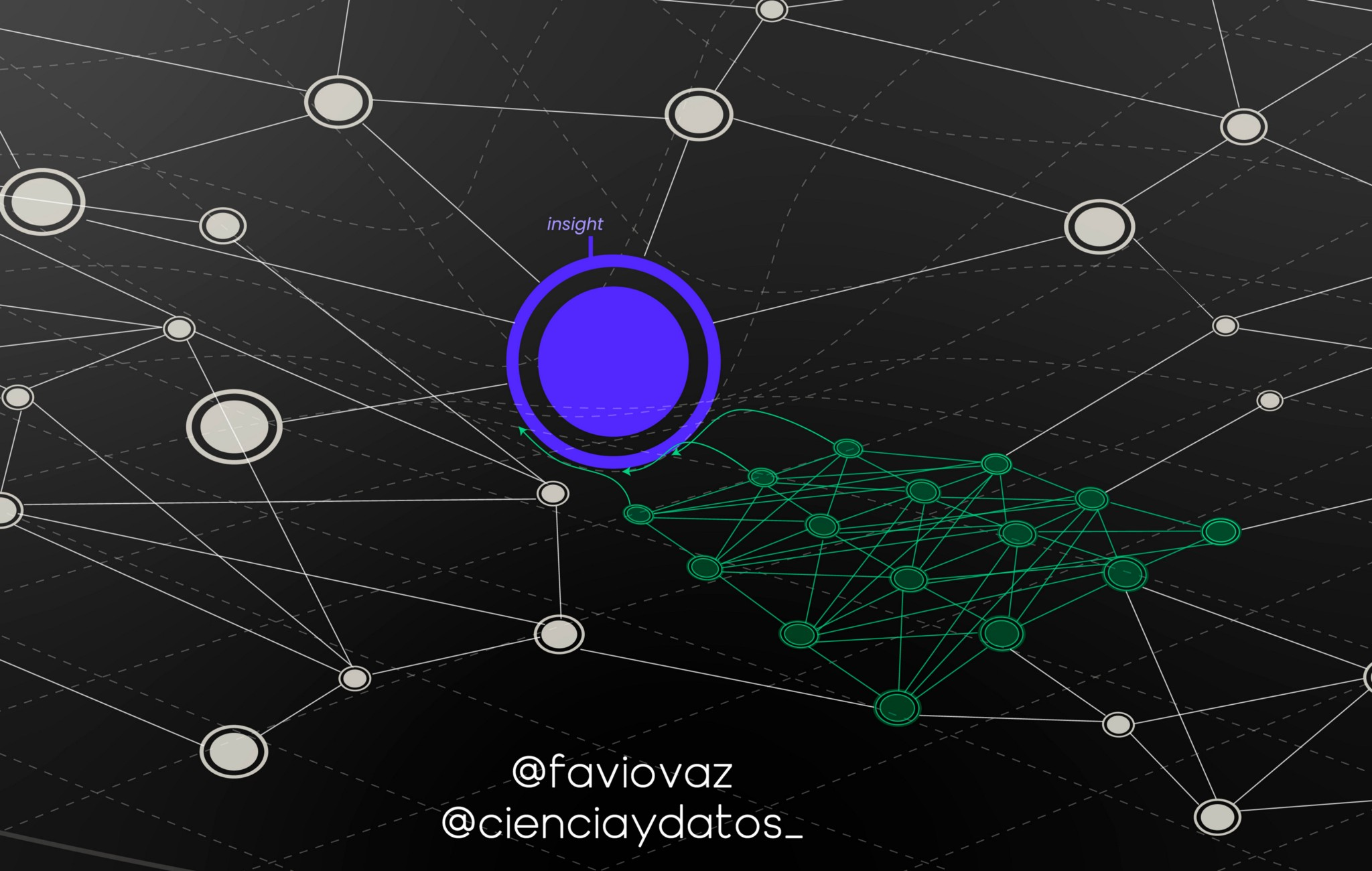总结

https://arxiv.org/pdf/1812.04202.pdf。